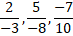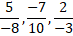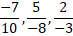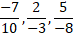# Quiz Discussion

The arrangement of rational numbers, -7/10, 5/-8, 2/-3  in ascending order is :

Course Name: Quantitative Aptitude

• 1]• 2]• 3]• 4]##### Solution
No Solution Present Yet

#### Top 5 Similar Quiz - Based On AI&ML

Quiz Recommendation System API Link - https://fresherbell-quiz-api.herokuapp.com/fresherbell_quiz_api

# Quiz
1
Discuss

The rational number for recurring decimal 0.125125.... is:

• 1]

63/487

• 2]

119/993

• 3]

125/999

• 4]

None of these

##### Solution
2
Discuss

58.621 - 13.829 - 7.302 - 1.214 = ?

• 1] 31.254
• 2] 35.272
• 3] 36.276
• 4] 37.281
• 5] None of these
##### Solution
3
Discuss

The fraction $$101\frac{{27}}{{100000}}$$   in decimal for is

• 1] .01027
• 2] .10127
• 3] 101.00027
• 4] 101.000027
##### Solution
4
Discuss

Which of the following numbers does not lie between 4/5 and 7/13 =?

• 1]

1/2

• 2]

2/3

• 3]

3/4

• 4]

5/7

##### Solution
5
Discuss

Solve $${\left( {\frac{{18}}{4}} \right)^2} \times \left( {\frac{{455}}{{19}}} \right) \div \left( {\frac{{61}}{{799}}} \right) = ?$$

• 1] 6320
• 2] 6400
• 3] 6350
• 4] 6430
• 5] 6490
##### Solution
6
Discuss

The rational numbers lying between 1/3 and 3/4 are :

• 1]

$$\frac{{117}}{{300}},\frac{{287}}{{400}}$$

• 2]

$$\frac{{95}}{{300}},\frac{{301}}{{400}}$$

• 3]

$$\frac{{99}}{{300}},\frac{{301}}{{400}}$$

• 4]

$$\frac{{97}}{{300}},\frac{{299}}{{500}}$$

##### Solution
7
Discuss

The correct expression of $$6.\overline {46}$$ in the fractional form is:

• 1]

646/99

• 2]

64640/1000

• 3]

640/100

• 4]

640/99

##### Solution
8
Discuss

The expression (11.98 × 11.98 + 11.98 × X + 0.02 × 0.02) will be a perfect square for X equal to:

• 1] 0.02
• 2] 0.2
• 3] 0.04
• 4] 0.4
##### Solution
9
Discuss

617 + 6.017 + 0.617 + 6.0017 = ?

• 1]

62.96357

• 2]

62963.57

• 3]

62.96357

• 4]

629.6357

##### Solution
10
Discuss

[(?)2 + (18)2] ÷ 125 = 3.56

• 1] 11
• 2] 12
• 3] 14
• 4] 15
• 5] None of these
# Quiz